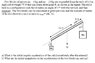# Moment of inertia Question

• Lord Dark

## Homework Statement

hello everyone ,, can someone tell me if I got the moment of inertia right or wrong of this question :
Two blocks of masses m1 = 5 kg and m2 = 15 kg are suspended from the ends of 1.5 m
rigid rod of weight 75 N that can rotate about point P, as shown in the figure. The rod is
held in a configuration such that it makes an angle of 37° with the vertical, and then
released. The two blocks can be considered as point particles and the moment of inertia
of the rod about its center of mass is I(com) = ML^2/12.

## The Attempt at a Solution

I=I(com)+M(L/3)^2+m1(2L/3)^2+m2(L/3)^2 = (7/36)ML^2+(m2L^2/9)+(4m1L^2/9),, my teacher told me that adding m2 and m1 is wrong ,, but what i don't get ,, in some questions we add them ,, so is he right or wrong ??

#### Attachments

•Q4.JPG
41.6 KB · Views: 351
Last edited:
And the figure shows what exactly?

Attach the figure.

sorry ,, forgot to add it

The masses are not rigidly attached to the stick, so don't include them when calculating the rotational inertia.

how come we don't include them ?? in the previous question (https://www.physicsforums.com/showthread.php?t=314630) we added them to the moment of inertia ,, in Fundamentals of physics book there is a question in Rotation chapter (11) Q:57P ,, in the answer .. it I*Alfa = (mL^2(1)+ mL^2(2))*alfa and it's almost the same as the question but without angle (it's horizontal) but I equaled : (mL^2(1)+ mL^2(2)) (the rod is mass less in this question)
By rigidly ,, you mean it fixed and can't be taken away ? ,, BTW some questions say (without the cord slipping on the pulley) does it mean it fixed ?

Last edited: